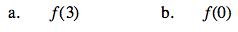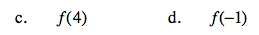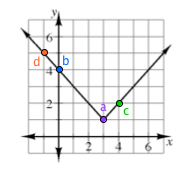### Home > CAAC > Chapter 12 > Lesson 12.1.3 > Problem12-26

12-26.
1.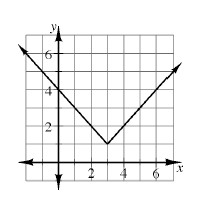Examine the graph of f(x) = | x − 3 | + 1 at right. Use the graph to find the values listed below. Homework Help ✎

1. f(3)

2. f(0)

3. f(4)

4. f(−1)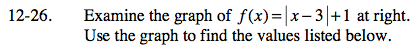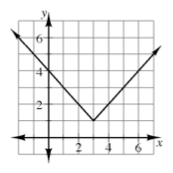The number in parentheses is the x-value that corresponds with a point on the function.
Find that point. Substitute the given x into the equation.